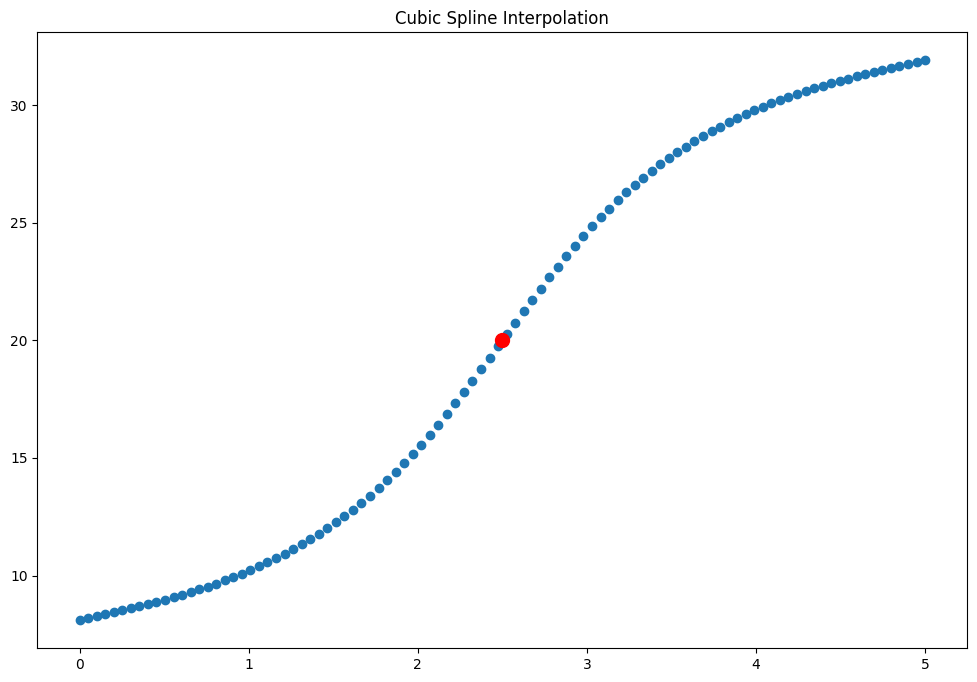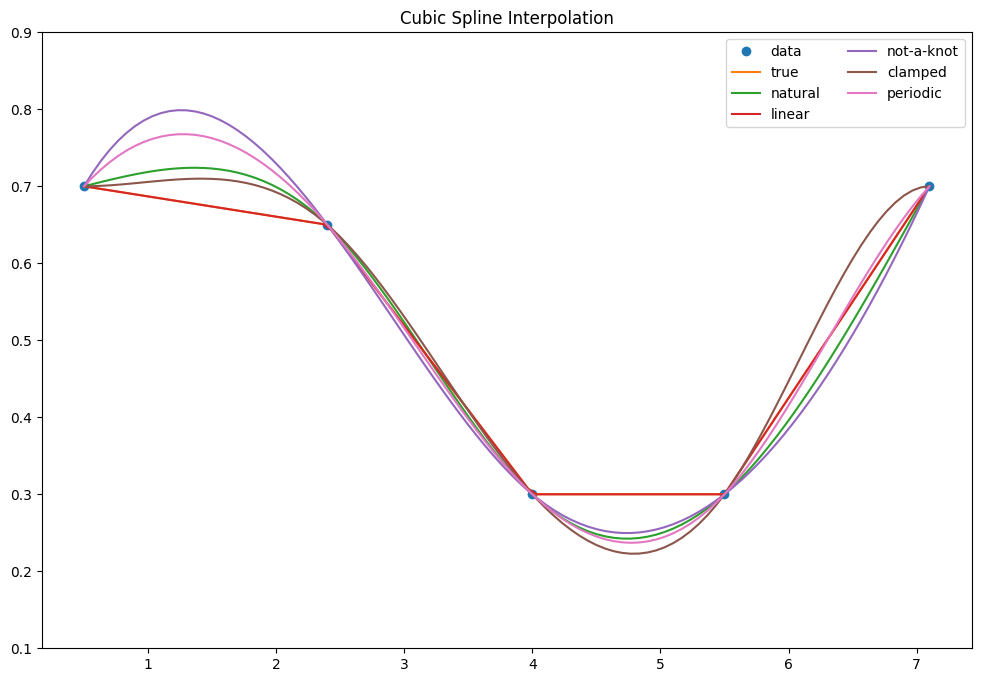# Spline Interpolation – How to find the polynomial curve to interpolate missing values

Spline interpolation is a special type of interpolation where a piecewise lower order polynomial called spline is fitted to the datapoints. That is, instead of fitting one higher order polynomial (as in polynomial interpolation), multiple lower order polynomials are fitted on smaller segments.

This can be implemented in Python.

You can do non-linear spline interpolation in Python using the pandas library by setting method='spline' in the interpolate() method of the series object.

By adjusting the order parameter, you can control the curviness of the splines.

## How to do Spline interpolation in Python?

First, let’s implement it with pandas using the interpolate method of a pandas series object. To use spline interpolation, you need to set the method to ‘spline’ and set the ‘order’ as well.

Let’s see an example based on the train fare example we saw in linear interpolation example.

import pandas as pd

fare = {'first_class':100,
'second_class':np.nan,
'third_class':60,
'open_class':20}

ser = pd.Series(fare)
ser

first_class     100.0
second_class      NaN
third_class      60.0
open_class       20.0
dtype: float64


We make sure the ‘x’ (index in this case) is in ascending order. So, we use ‘reset_index()’ method.

ser.reset_index().interpolate(method='spline', order=2)

index 0
0 first_class 100.000000
1 second_class 86.666667
2 third_class 60.000000
3 open_class 20.000000

What if you have the data as a Dataframe?

Works as well. But take care of the index.

# apply interpolate on a pandas dataframe.
data = pd.DataFrame(ser,columns=['Fare'])
data.reset_index().interpolate(method='spline', order=2)

index Fare
0 first_class 100.000000
1 second_class 86.666667
2 third_class 60.000000
3 open_class 20.000000

## Cubic spline interpolation using scipy

from scipy.interpolate import CubicSpline
import numpy as np

X = [0, 1, 2, 3, 4, 5]
Y = [8.1, 10.2, 15.4, 24.6, 29.8, 31.9]
x = 2.5

# make sure X is in ascending order
def make_ascending(X,Y):
if np.any(np.diff(X) < 0):
sorted_index = np.argsort(X).astype(int)
X = np.array(X)[sorted_index]
Y = np.array(Y)[sorted_index]
return X, Y

X, Y = make_ascending(X,Y)

# learn the CubicSpline function
f = CubicSpline(X, Y, bc_type='natural')

# Predict Y given x
f(x)

array(20.)


Let’s plot how the entire function will look like.

from scipy.interpolate import CubicSpline
import numpy as np
import matplotlib.pyplot as plt

X = [0, 1, 2, 3, 4, 5]
Y = [8.1, 10.2, 15.4, 24.6, 29.8, 31.9]

# Make ascending
X, Y = make_ascending(X,Y)

# Learn the cubic spline function
f = CubicSpline(X, Y, bc_type='natural')

# predict for the entire range of X
x = np.linspace(np.min(X), np.max(X), 100)
y = f(x)


Plot it

# Plot
plt.scatter(x, y)
plt.scatter(x=2.5, y=f(2.5), c='red', s=100)
plt.title('Cubic Spline Interpolation')
plt.show()## Comparing linear interpolation and various cubic spline interpolation

There are multiple methods within CubicSpline function from scipy. Let’s plot how they fit the data

# imports
import matplotlib.pyplot as plt
import numpy as np
from scipy.interpolate import CubicSpline, interp1d
plt.rcParams['figure.figsize'] =(12,8)

x = [.5, 2.4, 4.0, 5.5, 7.1]
y = [.7, .65, .3, .3, .7]

# apply cubic spline and linear interpolation
cs = CubicSpline(x, y, bc_type='natural')
cs_nak = CubicSpline(x, y, bc_type='not-a-knot')
cs_per = CubicSpline(x, y, bc_type='periodic')
cs_clm = CubicSpline(x, y, bc_type='clamped')
linear_int = interp1d(x,y)

# generate all possible X
X = np.linspace(np.min(x), np.max(x), 100)

# plot data
plt.plot(x, y, 'o', label='data')
plt.plot(x, y, label='true')

# Natural spline
plt.plot(X, cs(X), label="natural")

# Linear
plt.plot(X, linear_int(X),  label="linear")

# Not a Knot
plt.plot(X, cs_nak(X), label="not-a-knot")

# Clamped
plt.plot(X, cs_clm(X), label="clamped")

# Periodic
plt.plot(X, cs_per(X), label="periodic")

# plot elements
plt.ylim(.1, .9)
plt.legend(loc='upper right', ncol=2)
plt.title('Cubic Spline Interpolation')
plt.show()## Similar Articles

### Logistic Regression – A Complete Tutorial With Examples in RCourse Preview

## Machine Learning A-Z™: Hands-On Python & R In Data Science

### Free Sample Videos:#### Machine Learning A-Z™: Hands-On Python & R In Data Science#### Machine Learning A-Z™: Hands-On Python & R In Data Science#### Machine Learning A-Z™: Hands-On Python & R In Data Science#### Machine Learning A-Z™: Hands-On Python & R In Data Science